# Section G.4. MULTISECTION COMPLEX FSF FREQUENCY RESPONSE

### G 4 MULTISECTION COMPLEX FSF FREQUENCY RESPONSE

The frequency response of a guaranteed-stable complex N-section FSF, when r < 1, is Hgs,cplx(z) with the z variable in Eq. (7-18) replaced by ejw, givingTo temporarily simplify our expressions, we let q = w – 2pk/N, giving

Equation G-23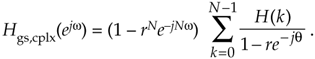Factoring out the half-angled exponentials, and accounting for the r factors, we have

Equation G-24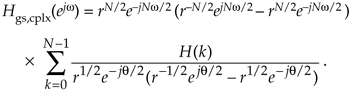Converting all the terms inside parentheses to exponentials (we'll see why in a moment), we have

Equation G-25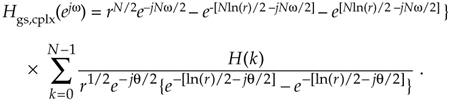The algebra gets a little messy here because our exponents have both real and imaginary parts. However, hyperbolic functions to the rescue. Recalling when a is a complex number, sinh(a) = (ea – e–a)/2, we have

Equation G-26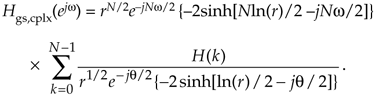Replacing angle q with w – 2pk/N, canceling the –2 factors, we have

Equation G-27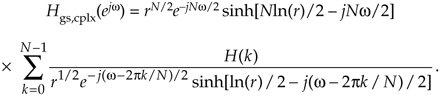Rearranging and combining terms we conclude with

Equation G-28(Whew! Now we see why this frequency response expression is not usually found in the literature.)

URL http://proquest.safaribooksonline.com/0131089897/app07lev1sec4AmazonPrev don't be afraid of buying books Next